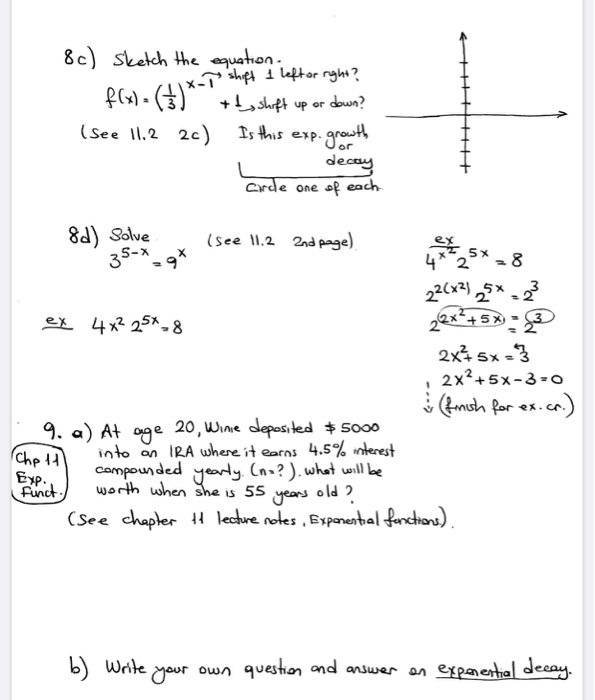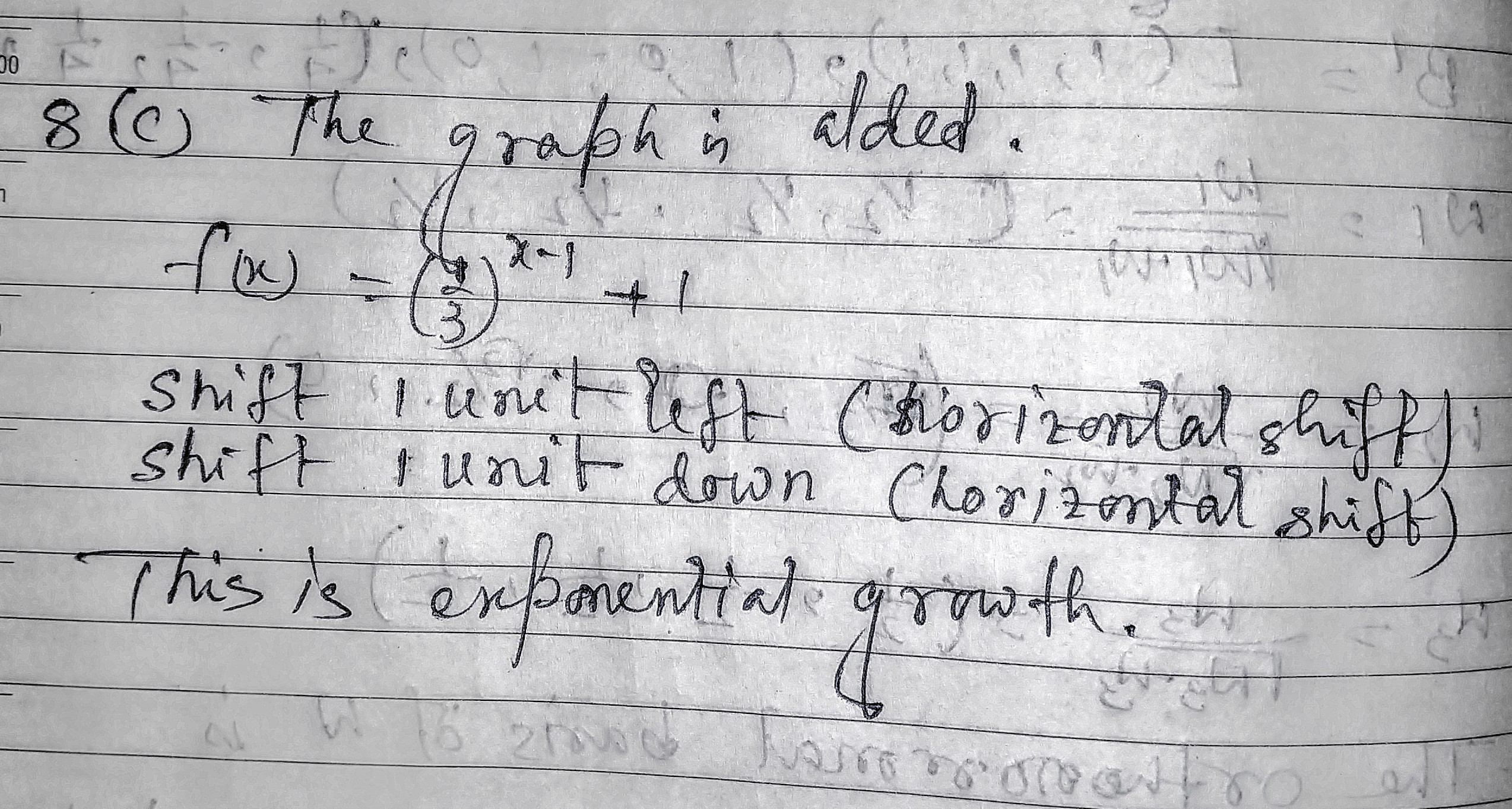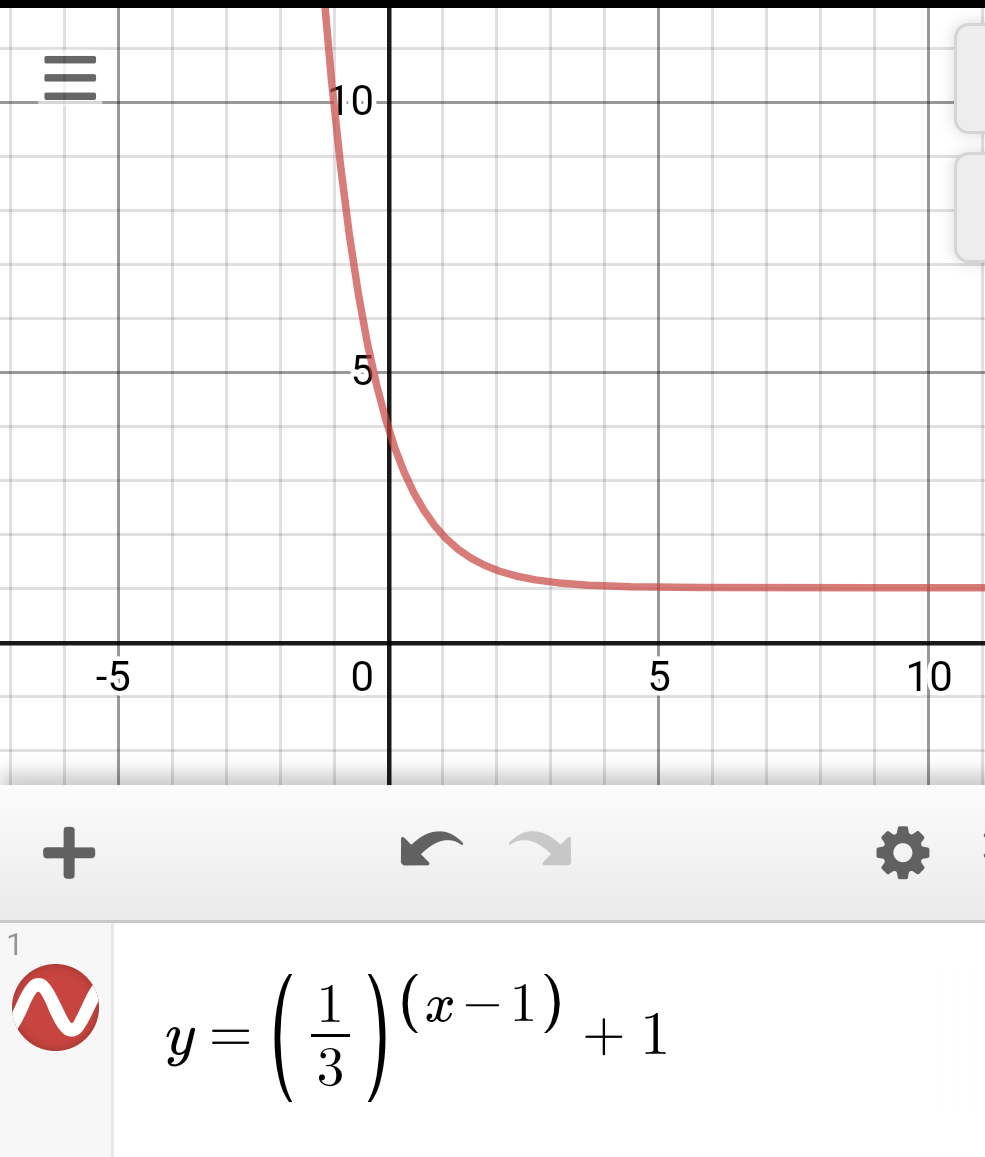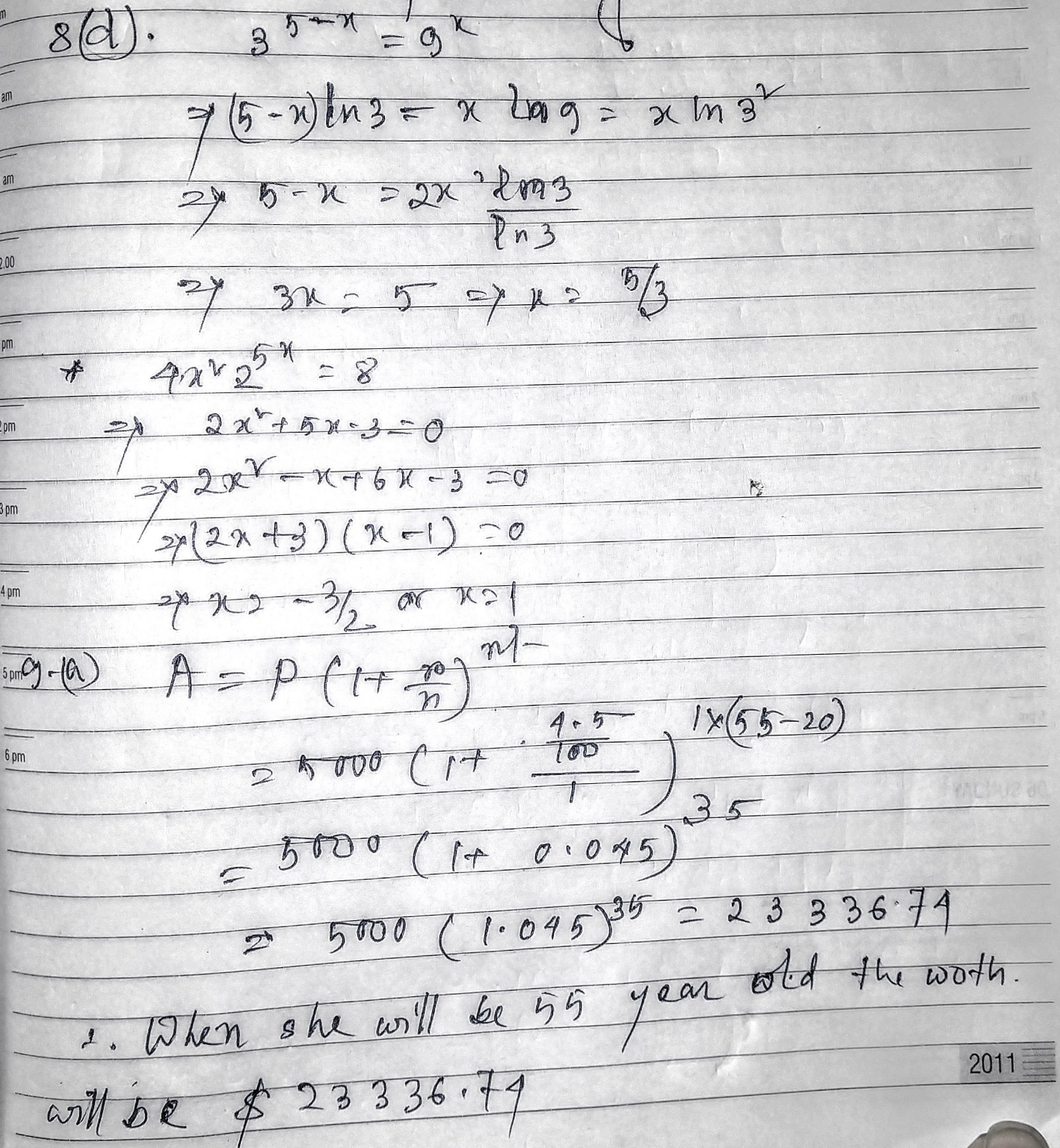# 8c) Sketch the equation. e shift I left or right? 1. f(x)= ( 3 ) +...8c) Sketch the equation. e shift I left or right? 1. f(x)= ( 3 ) + t shift up or down? (See 11.2 2c) Is this exp.growth decay Corde one of each 8d) Solve (see 11.2 2nd basel 35-*_ 22(x2),5* -g? ex 4x² 25+ 8 212x+583 2x+ 5x + 3 , 2x2+5X-3-0. (finish for ex. cr.) 9. a) At ouge 20, Winie deposited \$ 5000 (Cheld into an IRA where it earns 4.5% interest 1 compounded yearly. (n=? ). what will be Exprint worth when she is 55 years old? (see chapter H lecture notes, Exponential functions), b) Write your own question and answer on exponential decay.#### Earn Coin

Coins can be redeemed for fabulous gifts.